## Example Questions

### Example Question #133 : Trigonometry

The value of a cosine is positive in which quadrants?

The 3rd only

The 1st and 4th

The 1st and 3rd

The 4th only

The 1st and 4th

Explanation:

The cosine is positive in the 1st and 4th quadrants and negative in 2nd and 3rd

### Example Question #134 : Trigonometry

Which of the following is equal to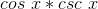?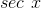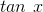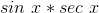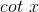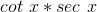Explanation:

Here, we use the SOHCAHTOA ratios and the fact that csc x = 1 / sin x.

Cosine x = adjacent side length / hypotenuse length

Cosecant x = 1 / sin x = hypotenuse / opposite

(Adjacent / hypotenuse) * (hypotenuse / opposite) = Adjacent / opposite = Cotangent x.

### Example Question #135 : Trigonometry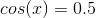andis betweenand.  What is the value of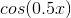?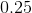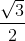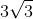Explanation:

Forto, we know that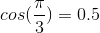.  So, the question asks, what is the value of, where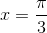.  Therefore, it is asking what the value of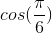is, which is.

### Example Question #136 : Trigonometry

To the nearest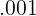, what is the cosine formed from the origin to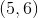? Assume counterclockwise rotation.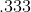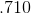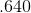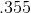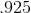Explanation:

If the point to be reached is, then we may envision a right triangle with sidesand, and hypotenuse. The Pythagorean Theorem tells us that, so we plug in and find that: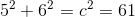Thus,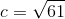Now, SOHCAHTOA tells us that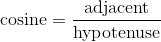, so we know that: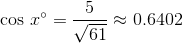Thus, our cosine is approximately.

### Example Question #137 : Trigonometry

Two drivers race to a finish line. Driver A drives northblocks, and eastblocks and crosses the goal. Driver B drives the shortest direct route between the two points. Relative to east, what is the cosine of the angle at which Driver B raced? Round to the nearest.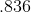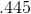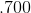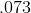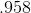Explanation:

If the point to be reached isblocks north andblocks east, then we may envision a right triangle with sidesand, and hypotenuse. The Pythagorean Theorem tells us that, so we plug in and find that: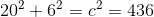Thus,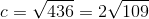Now, SOHCAHTOA tells us that, so we know that: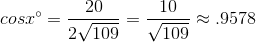Thus, our cosine is approximately.